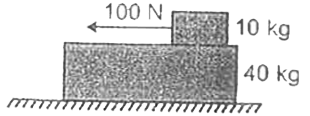Question

# A $40kg$ slab rests on a frictionless floor. A $10kg$ block rests on top of the slab. The coefficient of kinetic friction between the block and slab is $0.4$. A horizontal force of $100N$ applied on $10kg$ block . Find the resulting acceleration of the slab.

Open in App
Solution

##Step 1: Given DataMass of the slab ${m}_{s}=40kg$Mass of the block ${m}_{b}=10kg$The coefficient of kinetic friction between the block and slab $\mu =0.4$The horizontal force of $F=100N$ on $-x$ direction.Step 2: Calculate the Common accelerationThe total mass of the system,$m={m}_{s}+{m}_{b}$$⇒m=40+10=50kg$Since acceleration is action on $-x$ direction, the pseudo force will be acting on $+x$ direction with a force of $50a$.$\therefore 50a=100$$⇒a=2m/{s}^{2}$Step 3: Check acceleration of slab and blockLet us consider both the blocks move with the same acceleration.Pseudo force on the slab ${f}_{s}={m}_{s}a=40×2=80N$Pseudo force on the block ${f}_{b}={m}_{b}a=10×2=20N$Again, the normal force $N=100N$Therefore, limiting force, ${f}_{L}=\mu N=0.4×100=40N$Since ${f}_{s}>{f}_{L}$, this is not the case here.Step 4: Calculate resultant acceleration of slabTherefore, the block and the slab do not move with the same acceleration.If ${a}_{s}$ is the acceleration of the slab, then$40{a}_{s}=40$${a}_{s}=1m/{s}^{2}$Hence, the resulting acceleration of the slab is $1m/{s}^{2}$.Suggest Corrections0